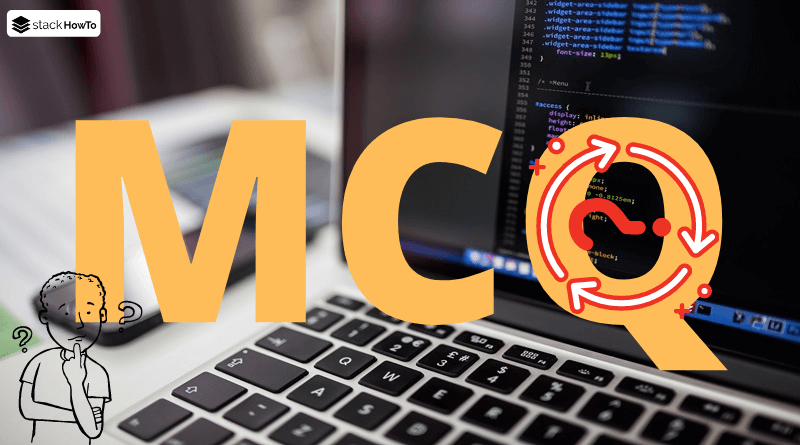MCQ

# Java 8 MCQ Online Test – Part 1

This collection of Java Multiple Choice Questions and Answers (MCQs): Quizzes & Practice Tests with Answer focuses on “Java 8”.

###### 1. Lambdas introduced in Java 8 allow us to process_____

A Data as code

B Code as data

C None of the above

D All the answers are true

B
Lambda expressions allow you to treat functionality as a method argument = code as data. This means that the code of your program that you write is also data that can be passed as an argument to another method and manipulated by a program.

Syntax of Lambda Expression:

`(argument-list) -> {body}`

###### 2. The newly introduced “Streams API” is available in which java 8 package?

A java.io.streams

B java.io.stream

C java.util.streams

D java.util.stream

D
Stream is a new abstract layer introduced in Java 8. Using stream, you can process data in a declarative manner similar to SQL statements.

A Clock

B Timer

C Time

D Date

A

###### 4. Lambda expressions in java 8 are based on _____

A Procedural programming

B Functional programming

C Data programming

D All the answers are true

B
Lambda expressions are like a way to support functional programming in Java. Functional programming is a paradigm for programming using expressions, declaring functions, passing functions as arguments, and using functions as instructions (called “expressions” in Java 8).

###### 5. How many methods exist in a functional interface in Java 8?

A 3

B 2

C 1

D 0

C
A functional interface is an interface that contains a single abstract method. They can have only one functionality. With Java 8, lambda expressions can be used to represent the instance of a functional interface.

###### 6. In Java 8 interfaces, methods can be ______

A default

B abstract

C Both A and B are true.

D None of the above

C

###### 7. Which of the following is not introduced with Java 8?

A API Stream

B Spliterator

C Lambda Expression

D Serialization

D
Serialization is not introduced with Java 8. It was introduced with an earlier version of Java.

A String

B Object

C Function

D void

C

###### 9. What is the new method introduced in java 8 to iterate through a collection?

A `List.for()`

B `StringList.forEach()`

C `foreach(String i : StringList)`

D `for(String i : StringList)`

B

###### 10. What are the two types of Streams proposed by Java 8?

A Random and synchronized

B Parallel and random

C Sequential and random

D Sequential and parallel

D
Sequential Stream and Parallel Stream are two types of Stream provided by java.

```Stream<Integer> sequential = list.stream();
Stream<Integer> parallel = list.parallelStream();```MCQPractice competitive and technical Multiple Choice Questions and Answers (MCQs) with simple and logical explanations to prepare for tests and interviews.Read More